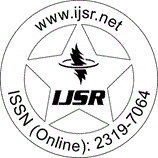International Journal of Science and Research (IJSR)
Call for Papers | Fully Refereed | Open Access | Double Blind Peer Reviewed

Downloads: 117 | Views: 168 | Weekly Hits: ⮙1 | Monthly Hits: ⮙1

Research Paper | Earth Science and Engineering | Indonesia | Volume 4 Issue 11, November 2015

# Developed Forward and Inverse Modelling of Vertical Electrical Sounding (VES) Using MATLAB Implementation

La Hamimu | Jamhir Safani | LO Ngkoimani

Abstract: We have implemented a MATLAB source code for VES forward modeling and its inversion using a genetic algorithm (GA) optimization technique. The codes presented here are applied to the Schlumberger electrode arrangement. In the forward modeling computation, we have developed code to generate theoretical apparent resistivity curves from a specified layered earth model. The input to this program consists of the number of layers, the layer resistivity and thickness. The output of this program is apparent resistivity versus electrode spacing incorporated in the inversion process as apparent resistivity data. For the inversion, we have developed a MATLAB code to invert (for layer resistivity and thickness) the apparent resistivity data by the genetic algorithm optimization technique. The code also has some function files involving the basic stages in the GA inversion. Our inversion procedure addressed calculates forward solutions from sets of random input, to find the apparent resistivity. Then, it evolves the models by better sets of inputs through processes that imitate natural mating, selection, crossover, and mutation in each generation. The aim of GA inversion is to find the best correlation between model and theoretical apparent resistivity curves. In this study, we present three synthetic examples that demonstrate the effectiveness and usefulness of this program. Our numerical modeling shows that the GA optimization technique can be applied for resolving layer parameters with reasonably low error values.

Keywords: Forward and inverse modelling, vertical electrical sounding, MATLAB implementation, genetic algorithm, apparent resistivity

Edition: Volume 4 Issue 11, November 2015,

Pages: 1592 - 1597